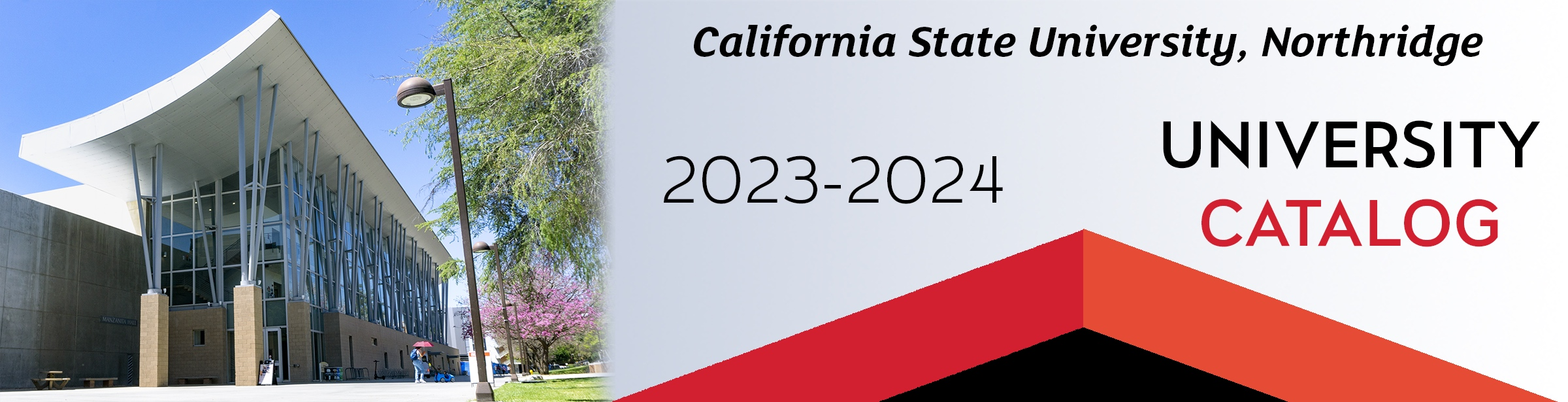## Course: ECE 309. Numerical Methods in Electrical Engineering (2)

Prerequisites: ECE 240; MATH 280 or ECE 280; ECE 206/L or COMP 110/L. This course includes numerical techniques implemented in MATLAB for the solution of problems in electrical and computer engineering. Topics covered include an introduction to MATLAB, number representation and error analysis, interpolation and curve-fitting, numerical solutions to systems of linear equations, root-finding, differentiation, integration, and basic statistics. Two 3-hour labs per week.

### ECE 309

Class NumberLocationDayTime